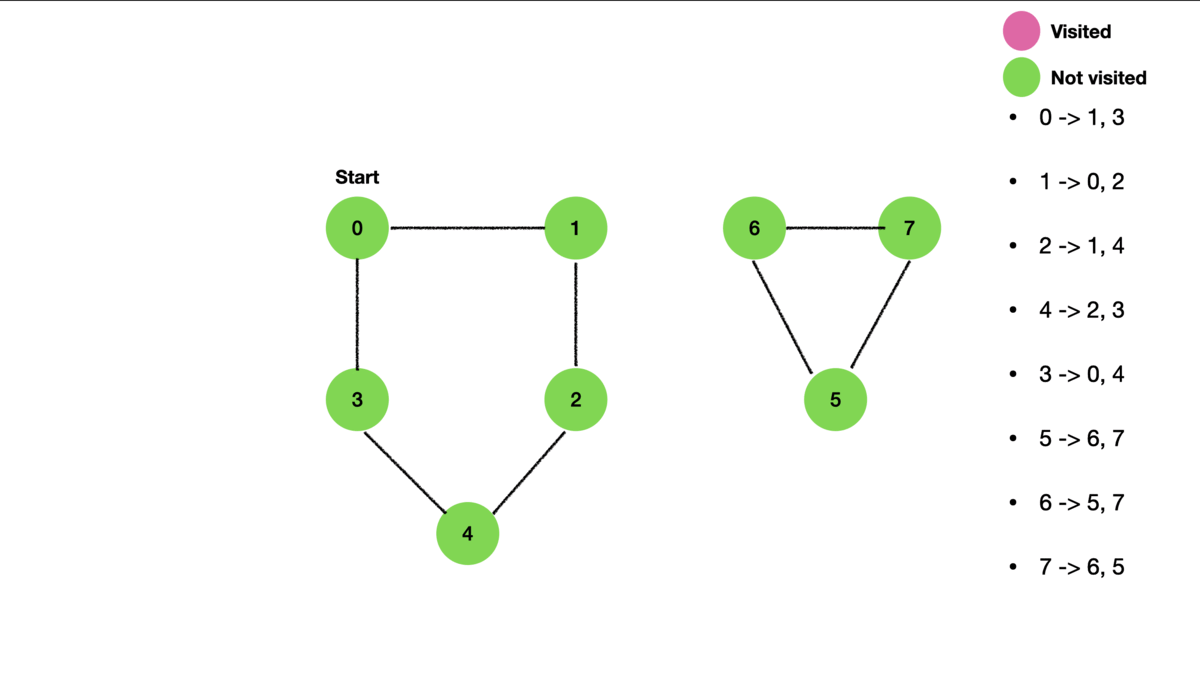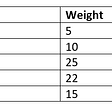# How to create a BST:

`class Node {   constructor (val) {      this.val = val;       this.left = null;       this.right = null;   }}`
`class BST {    constructor (val) {     this.root = null    }}`
`class BST {    constructor (val) {      this.root = null    }   insert (val) {         }}`

# How to insert into a BST

1. Create a variable newNode that takes in a new Node from the previous class.
2. If there is no root, then given the value of new node, assign the new node to be the root.
3. Create a variable called current to be equal to the root.
4. While true, if the current value is equal to the value given in the parameter, return undefined.
5. If the value is less than the current value, and if the current.left is null, assign the current.left equal to newNode. Once this is assigned, reassign current to be current.left (aka null)
6. If the value is greater than the current value, and if the current.right is null, assign the current.right = to the newNode. Once this is assigned, reassign the current to be current.right (aka null)
`class BST {   constructor() {   this.root = null   }   insert(val){   let newNode = new Node(val)   if (!this.root) {   this.root = newNode   return this;  }   let current = this.root;   while (true){   if (current.val === val) return undefined;     if (val < current.val) {         if (current.left === null) {            current.left = newNode            return this;         }      current = current.left      } else {         if (current.right === null) {         current.right = newNode          return this;        }     current = current.right     }   }}`
`let newTree = new BST()`
`newTree.insert(5)newTree.insert(6)newTree.insert(7)newTree.insert(3)`
`    5  /  \3      6         \           7`

# LeetCode 653

`const traverseDFS = (tree, k) => {    let hash = new Set() // o (1)    let stack = [tree]    while (stack.length > 0) {   // o (n)       let current = stack.pop()              if (hash.has(current.val)) {         return true       }         hash.add(k - current.val)       if (current.left) stack.push(current.left)        if (current.right) stack.push(current.right)   }   return false }`

## More from Crystal Villanueva

Flatiron student, word nerd and otter enthusiast

Love podcasts or audiobooks? Learn on the go with our new app.

## The Rare <audio> HTML5 Tag## Setting up Phaser 3 with Webpack and NodeJS — Part II## Node.js Mentorship Working Group## Integrating Firebase Auth into Strapi.## Lazy Evaluation in JavaScript## Building a GitHub Repo Explorer with React and Elasticsearch## Middleware, middlewhat? API essential## Using Async Await Inside React’s useEffect() Hook## Crystal Villanueva

Flatiron student, word nerd and otter enthusiast

## Anagrams in Dictionary## Graph Algorithm — Depth First Search## Knapsack problem using greedy method